Documentation

fft

Fast Fourier transform

Description

example

Y = fft(X) computes the discrete Fourier transform (DFT) of X using a fast Fourier transform (FFT) algorithm.

• If X is a vector, then fft(X) returns the Fourier transform of the vector.

• If X is a matrix, then fft(X) treats the columns of X as vectors and returns the Fourier transform of each column.

• If X is a multidimensional array, then fft(X) treats the values along the first array dimension whose size does not equal 1 as vectors and returns the Fourier transform of each vector.

example

Y = fft(X,n) returns the n-point DFT. If no value is specified, Y is the same size as X.

• If X is a vector and the length of X is less than n, then X is padded with trailing zeros to length n.

• If X is a vector and the length of X is greater than n, then X is truncated to length n.

• If X is a matrix, then each column is treated as in the vector case.

• If X is a multidimensional array, then the first array dimension whose size does not equal 1 is treated as in the vector case.

example

Y = fft(X,n,dim) returns the Fourier transform along the dimension dim. For example, if X is a matrix, then fft(X,n,2) returns the n-point Fourier transform of each row.

Examples

collapse all

Use Fourier transforms to find the frequency components of a signal buried in noise.

Specify the parameters of a signal with a sampling frequency of 1 kHz and a signal duration of 1.5 seconds.

Fs = 1000;            % Sampling frequency
T = 1/Fs;             % Sampling period
L = 1500;             % Length of signal
t = (0:L-1)*T;        % Time vector

Form a signal containing a 50 Hz sinusoid of amplitude 0.7 and a 120 Hz sinusoid of amplitude 1.

S = 0.7*sin(2*pi*50*t) + sin(2*pi*120*t);

Corrupt the signal with zero-mean white noise with a variance of 4.

X = S + 2*randn(size(t));

Plot the noisy signal in the time domain. It is difficult to identify the frequency components by looking at the signal X(t).

plot(1000*t(1:50),X(1:50))
title('Signal Corrupted with Zero-Mean Random Noise')
xlabel('t (milliseconds)')
ylabel('X(t)')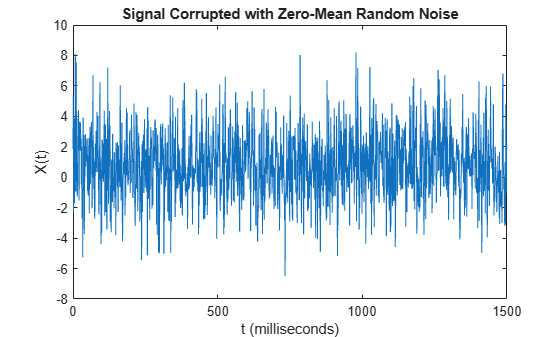Compute the Fourier transform of the signal.

Y = fft(X);

Compute the two-sided spectrum P2. Then compute the single-sided spectrum P1 based on P2 and the even-valued signal length L.

P2 = abs(Y/L);
P1 = P2(1:L/2+1);
P1(2:end-1) = 2*P1(2:end-1);

Define the frequency domain f and plot the single-sided amplitude spectrum P1. The amplitudes are not exactly at 0.7 and 1, as expected, because of the added noise. On average, longer signals produce better frequency approximations.

f = Fs*(0:(L/2))/L;
plot(f,P1)
title('Single-Sided Amplitude Spectrum of X(t)')
xlabel('f (Hz)')
ylabel('|P1(f)|')Now, take the Fourier transform of the original, uncorrupted signal and retrieve the exact amplitudes, 0.7 and 1.0.

Y = fft(S);
P2 = abs(Y/L);
P1 = P2(1:L/2+1);
P1(2:end-1) = 2*P1(2:end-1);

plot(f,P1)
title('Single-Sided Amplitude Spectrum of S(t)')
xlabel('f (Hz)')
ylabel('|P1(f)|')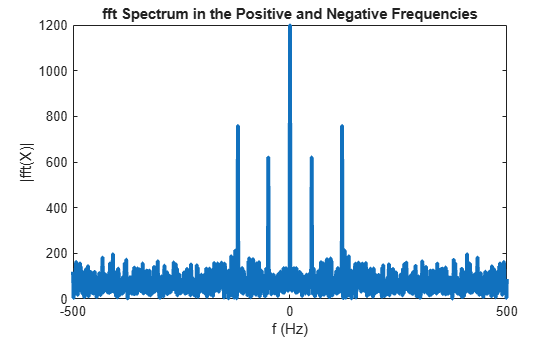Convert a Gaussian pulse from the time domain to the frequency domain.

Define signal parameters and a Gaussian pulse, X.

Fs = 100;           % Sampling frequency
t = -0.5:1/Fs:0.5;  % Time vector
L = length(t);      % Signal length

X = 1/(4*sqrt(2*pi*0.01))*(exp(-t.^2/(2*0.01)));

Plot the pulse in the time domain.

plot(t,X)
title('Gaussian Pulse in Time Domain')
xlabel('Time (t)')
ylabel('X(t)')To use the fft function to convert the signal to the frequency domain, first identify a new input length that is the next power of 2 from the original signal length. This will pad the signal X with trailing zeros in order to improve the performance of fft.

n = 2^nextpow2(L);

Convert the Gaussian pulse to the frequency domain.

Y = fft(X,n);

Define the frequency domain and plot the unique frequencies.

f = Fs*(0:(n/2))/n;
P = abs(Y/n);

plot(f,P(1:n/2+1))
title('Gaussian Pulse in Frequency Domain')
xlabel('Frequency (f)')
ylabel('|P(f)|')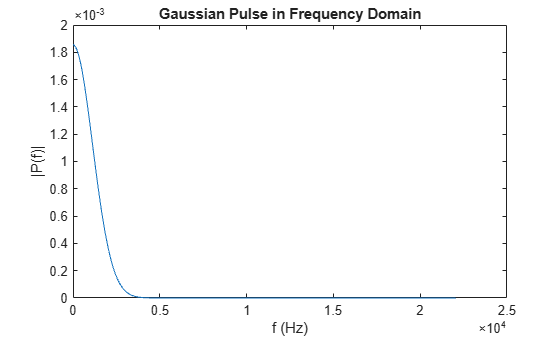Compare cosine waves in the time domain and the frequency domain.

Specify the parameters of a signal with a sampling frequency of 1kHz and a signal duration of 1 second.

Fs = 1000;                    % Sampling frequency
T = 1/Fs;                     % Sampling period
L = 1000;                     % Length of signal
t = (0:L-1)*T;                % Time vector

Create a matrix where each row represents a cosine wave with scaled frequency. The result, X, is a 3-by-1000 matrix. The first row has a wave frequency of 50, the second row has a wave frequency of 150, and the third row has a wave frequency of 300.

x1 = cos(2*pi*50*t);          % First row wave
x2 = cos(2*pi*150*t);         % Second row wave
x3 = cos(2*pi*300*t);         % Third row wave

X = [x1; x2; x3];

Plot the first 100 entries from each row of X in a single figure in order and compare their frequencies.

for i = 1:3
subplot(3,1,i)
plot(t(1:100),X(i,1:100))
title(['Row ',num2str(i),' in the Time Domain'])
end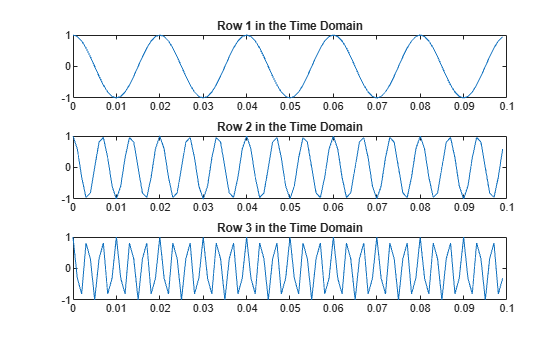For algorithm performance purposes, fft allows you to pad the input with trailing zeros. In this case, pad each row of X with zeros so that the length of each row is the next higher power of 2 from the current length. Define the new length using the nextpow2 function.

n = 2^nextpow2(L);

Specify the dim argument to use fft along the rows of X, that is, for each signal.

dim = 2;

Compute the Fourier transform of the signals.

Y = fft(X,n,dim);

Calculate the double-sided spectrum and single-sided spectrum of each signal.

P2 = abs(Y/L);
P1 = P2(:,1:n/2+1);
P1(:,2:end-1) = 2*P1(:,2:end-1);

In the frequency domain, plot the single-sided amplitude spectrum for each row in a single figure.

for i=1:3
subplot(3,1,i)
plot(0:(Fs/n):(Fs/2-Fs/n),P1(i,1:n/2))
title(['Row ',num2str(i),' in the Frequency Domain'])
end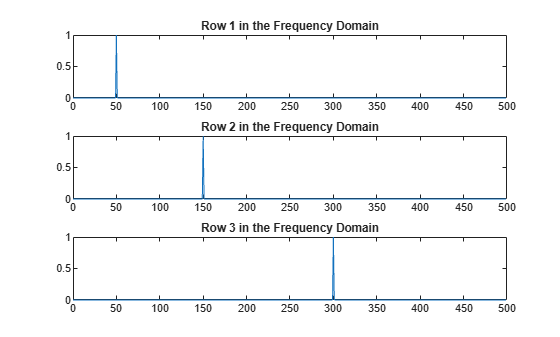Input Arguments

collapse all

Input array, specified as a vector, matrix, or multidimensional array.

If X is an empty 0-by-0 matrix, then fft(X) returns an empty 0-by-0 matrix.

Data Types: double | single | int8 | int16 | int32 | uint8 | uint16 | uint32 | logical
Complex Number Support: Yes

Transform length, specified as [] or a nonnegative integer scalar. Specifying a positive integer scalar for the transform length can increase the performance of fft. The length is typically specified as a power of 2 or a value that can be factored into a product of small prime numbers. If n is less than the length of the signal, then fft ignores the remaining signal values past the nth entry and returns the truncated result. If n is 0, then fft returns an empty matrix.

Example: n = 2^nextpow2(size(X,1))

Data Types: double | single | int8 | int16 | int32 | uint8 | uint16 | uint32 | logical

Dimension to operate along, specified as a positive integer scalar. If no value is specified, then the default is the first array dimension whose size does not equal 1.

• fft(X,[],1) operates along the columns of X and returns the Fourier transform of each column.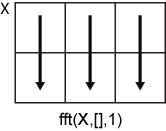• fft(X,[],2) operates along the rows of X and returns the Fourier transform of each row.If dim is greater than ndims(X), then fft(X,[],dim) returns X. When n is specified, fft(X,n,dim) pads or truncates X to length n along dimension dim.

Data Types: double | single | int8 | int16 | int32 | uint8 | uint16 | uint32 | logical

Output Arguments

collapse all

Frequency domain representation returned as a vector, matrix, or multidimensional array.

If X is of type single, then fft natively computes in single precision, and Y is also of type single. Otherwise, Y is returned as type double.

The size of Y is as follows:

• For Y = fft(X) or Y = fft(X,[],dim), the size of Y is equal to the size of X.

• For Y = fft(X,n,dim), the value of size(Y,dim) is equal to n, while the size of all other dimensions remains as in X.

If X is real, then Y is conjugate symmetric, and the number of unique points in Y is ceil((n+1)/2).

Data Types: double | single

collapse all

Discrete Fourier Transform of Vector

Y = fft(X) and X = ifft(Y) implement the Fourier transform and inverse Fourier transform, respectively. For X and Y of length n, these transforms are defined as follows:

$\begin{array}{l}Y\left(k\right)=\sum _{j=1}^{n}X\left(j\right)\text{\hspace{0.17em}}{W}_{n}^{\left(j-1\right)\text{​}\left(k-1\right)}\\ X\left(j\right)=\frac{1}{n}\sum _{k=1}^{n}Y\left(k\right)\text{\hspace{0.17em}}{W}_{n}{}^{-\left(j-1\right)\text{​}\left(k-1\right)},\end{array}$

where

${W}_{n}={e}^{\left(-2\pi i\right)/n}$

is one of n roots of unity.

Tips

• The execution time for fft depends on the length of the transform. Transform lengths that have only small prime factors are significantly faster than those that are prime or have large prime factors.

• For most values of n, real-input DFTs require roughly half the computation time of complex-input DFTs. However, when n has large prime factors, there is little or no speed difference.

• You can potentially increase the speed of fft using the utility function, fftw. This function controls the optimization of the algorithm used to compute an FFT of a particular size and dimension.

Algorithms

The FFT functions (fft, fft2, fftn, ifft, ifft2, ifftn) are based on a library called FFTW  .

 Frigo, M., and S. G. Johnson. “FFTW: An Adaptive Software Architecture for the FFT.” Proceedings of the International Conference on Acoustics, Speech, and Signal Processing. Vol. 3, 1998, pp. 1381-1384.